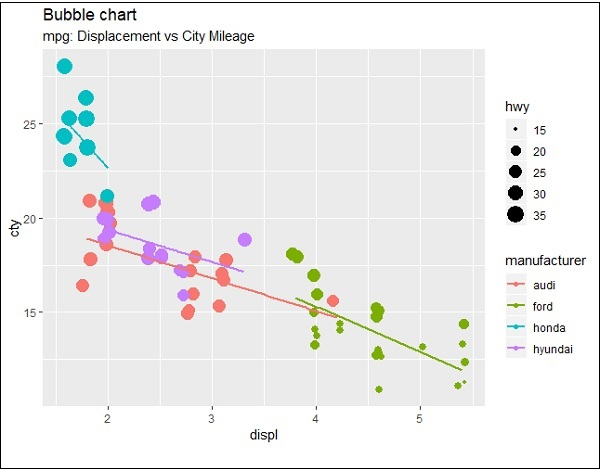# ggplot2 - Bubble Plots & Count Charts

Bubble plots are nothing but bubble charts which is basically a scatter plot with a third numeric variable used for circle size. In this chapter, we will focus on creation of bar count plot and histogram count plots which is considered as replica of bubble plots.

Following steps are used to create bubble plots and count charts with mentioned package −

## Understanding Dataset

Load the respective package and the required dataset to create the bubble plots and count charts.

```> # Load ggplot
> library(ggplot2)
>
> data(mpg)
# A tibble: 6 x 11
manufacturer  model  displ  year  cyl  trans      drv   cty  hwy  fl  class
<chr> <chr> <dbl> <int> <int> <chr> <chr> <int> <int> <chr> <chr>
1 audi        a4     1.8    1999  4    auto(l5)   f     18   29   p   compa~
2 audi        a4     1.8    1999  4    manual(m5) f     21   29   p   compa~
3 audi        a4     2      2008  4    manual(m6) f     20   31   p   compa~
4 audi        a4     2      2008  4    auto(av)   f     21   30   p   compa~
5 audi        a4     2.8    1999  6    auto(l5)   f     16   26   p   compa~
6 audi        a4     2.8    1999  6    manual(m5) f     18   26   p   compa~
```

The bar count plot can be created using the following command −

```> # A bar count plot
> p <- ggplot(mpg, aes(x=factor(cyl)))+
+ geom_bar(stat="count")
> p
```## Analysis with Histograms

The histogram count plot can be created using the following command −

```> # A historgram count plot
> ggplot(data=mpg, aes(x=hwy)) +
+    geom_histogram( col="red",
+       fill="green",
+       alpha = .2,
+       binwidth = 5)
```## Bubble Charts

Now let us create the most basic bubble plot with the required attributes of increasing the dimension of points mentioned in scattered plot.

```ggplot(mpg, aes(x=cty, y=hwy, size = pop)) +geom_point(alpha=0.7)
```The plot describes the nature of manufacturers which is included in legend format. The values represented include various dimensions of “hwy” attribute.